## Wednesday, May 17, 2017

### Looking at the Platinum dataset

A recent paper out of the ZBH in Hamburg published a new set of ligand structures from the PDB to be used for benchmarking conformational analysis methods: http://pubs.acs.org/doi/abs/10.1021/acs.jcim.6b00613 The dataset is available here: http://www.zbh.uni-hamburg.de/?id=628 Along with the paper the authors presented results from multiple conformation generation tools, including the RDKit. Sereina Riniker's ETKDG implementation (http://pubs.acs.org/doi/abs/10.1021/acs.jcim.5b00654) in the RDKit ended up doing quite well relative to the other approaches. That, of course, made me quite happy.
I hadn't done anything with the Platinum dataset at all yet, but an exchange with David Koes on Twitter - https://twitter.com/david_koes/status/862831249617862656 - prompted me to take a look. It's an interesting one to look at from the "predicting bioactive conformations" perspective since it has >4500 PDB ligands. In the ETKDG paper we only looked at 238 PDB structures; that paper also included 1290 CSD structures.
In :
```from collections import defaultdict
import numpy as np
from rdkit import Chem
from rdkit.Chem import AllChem
from rdkit.Chem.Draw import IPythonConsole
IPythonConsole.ipython_3d=True
%pylab inline
```
```Populating the interactive namespace from numpy and matplotlib
```
```/home/glandrum/anaconda3/envs/py36/lib/python3.6/site-packages/IPython/core/magics/pylab.py:160: UserWarning: pylab import has clobbered these variables: ['indices']
`%matplotlib` prevents importing * from pylab and numpy
"\n`%matplotlib` prevents importing * from pylab and numpy"
```
Read the molecules in and assign atomic chirality based on the structures provided.
In :
```ms = [x for x in Chem.SDMolSupplier('../data/platinum_dataset_2017_01.sdf',removeHs=False)]
# Assign atomic chirality based on the structures:
for m in ms: Chem.AssignAtomChiralTagsFromStructure(m)
len(ms)
```
Out:
`4548`

# Conformation generation¶

Start by generating 50 conformations via ETKDG
In :
```import time
tms = [Chem.Mol(x) for x in ms]
ps = AllChem.ETKDG()
ps.pruneRmsThresh=0.5
ps.numThreads=0
ts = []
for i,m in enumerate(tms):
t1 = time.time()
AllChem.EmbedMultipleConfs(m,50,ps)
t2=time.time()
ts.append((t2-t1))
if not (i%50): print("done %d"%i)
```
```done 0
done 50
done 100
done 150
```
`  ... `
```done 4350
done 4400
done 4450
done 4500
```
Repeat the conformation generation using standard DG
In :
```tms2 = [Chem.Mol(x) for x in ms]
ps = AllChem.ETKDG()
ps.useBasicKnowledge = False
ps.useExpTorsionAnglePrefs = False
ps.pruneRmsThresh=0.5
ps.numThreads=0
ts2 = []
for i,m in enumerate(tms2):
t1 = time.time()
AllChem.EmbedMultipleConfs(m,50,ps)
t2=time.time()
ts2.append((t2-t1))
if not (i%50): print("done %d"%i)
```
```done 0
done 50
done 100
done 150```
` ...`
`done 4350`
```done 4400
done 4450
done 4500
```

# RMSD calculation¶

For the RMS analysis we want molecules without Hs:
In :
```ms_noh = [Chem.RemoveHs(m) for m in ms]
tms_noh = [Chem.RemoveHs(m) for m in tms]
tms2_noh = [Chem.RemoveHs(m) for m in tms2]
```
In :
```import pickle
with open('../data/platinum_dataset_2017_01.chiral_confs.pkl','wb+') as outf:
pickle.dump((ms_noh,tms_noh,tms2_noh,ts,ts2),outf)
```
Now generate RMS values to the crystal structure for 1, 5, 20, 25, and 50 conformer ensembles.
In :
```# ETKDG
rmsvs = defaultdict(list)
for m,tm in zip(ms_noh,tms_noh):
best = 1e8
cids = [x.GetId() for x in tm.GetConformers()]
for i,cid in enumerate(cids):
rms = AllChem.GetBestRMS(m,tm,probeConfId=cid)
best = min(rms,best)
if not i:
best_1 = best
if i<5:
best_5 = best
if i<20:
best_20 = best
if i<25:
best_25 = best
rmsvs.append(best)
rmsvs.append(best_1)
rmsvs.append(best_5)
rmsvs.append(best_20)
rmsvs.append(best_25)
# DG
rmsvs2 = defaultdict(list)
for m,tm in zip(ms_noh,tms2_noh):
best = 1e8
cids = [x.GetId() for x in tm.GetConformers()]
for i,cid in enumerate(cids):
rms = AllChem.GetBestRMS(m,tm,probeConfId=cid)
best = min(rms,best)
if not i:
best_1 = best
if i<5:
best_5 = best
if i<20:
best_20 = best
if i<25:
best_25 = best
rmsvs2.append(best)
rmsvs2.append(best_1)
rmsvs2.append(best_5)
rmsvs2.append(best_20)
rmsvs2.append(best_25)
```
There are a couple molecules where we didn't manage to generate conformations:
In :
```len([1 for x in rmsvs if x>1000]),len([1 for x in rmsvs2 if x>1000])
```
Out:
`(2, 1)`
Let's look at those:
In :
```[i for i,x in enumerate(rmsvs) if x > 1000],[i for i,x in enumerate(rmsvs2) if x > 1000]
```
Out:
`([1641, 2708], )`
In :
```from rdkit.Chem import Draw
indices = [i for i,x in enumerate(rmsvs) if x > 1000]
badms = [Draw.PrepareMolForDrawing(ms_noh[x],forceCoords=True) for x in indices]
legends = [ms[x].GetProp("_Name") for x in indices]
Draw.MolsToGridImage(badms,legends=legends)
```
Out: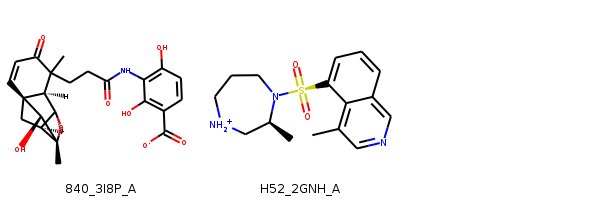I'm surprised that there were problems with the second structure (H52). It doesn't look like it should be that hard to embed, so that's something to look into. The first structure (840) has multiple specified chiral centers in a highly constrained fused ring system, so it's not horribly surprising to encounter problems.

# Looking at RMSD values¶

In :
```def values_there(seq,threshold=1000):
return [x for x in seq if x<threshold]
```
Let's look at histograms of RMS values
In :
```figsize(18,6)
hist([values_there(rmsvs[x]) for x in sorted(rmsvs)],bins=20,label=[x for x in sorted(rmsvs)]);
legend();
title('ETKDG');
xlabel('RMSD')
ylabel('Number of structures');
```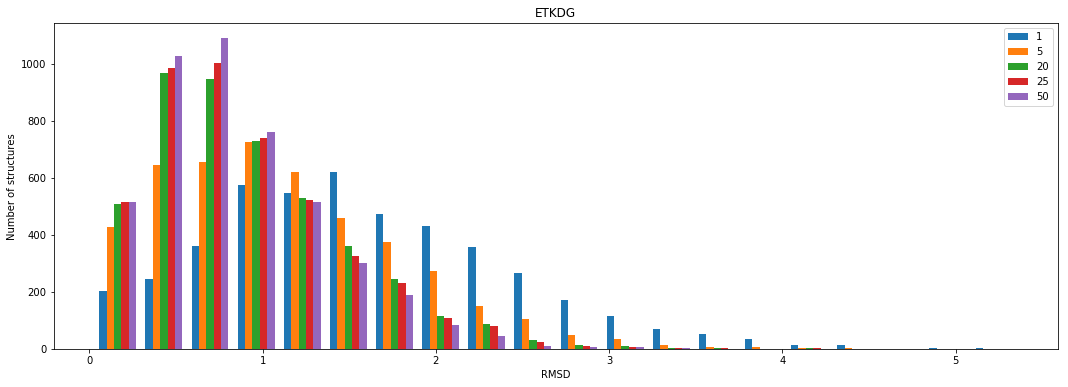In :
```figsize(18,6)
hist([values_there(rmsvs2[x]) for x in sorted(rmsvs2)],bins=20,label=[x for x in sorted(rmsvs2)]);
legend();
title('default DG');
xlabel('RMSD')
ylabel('Number of structures');
```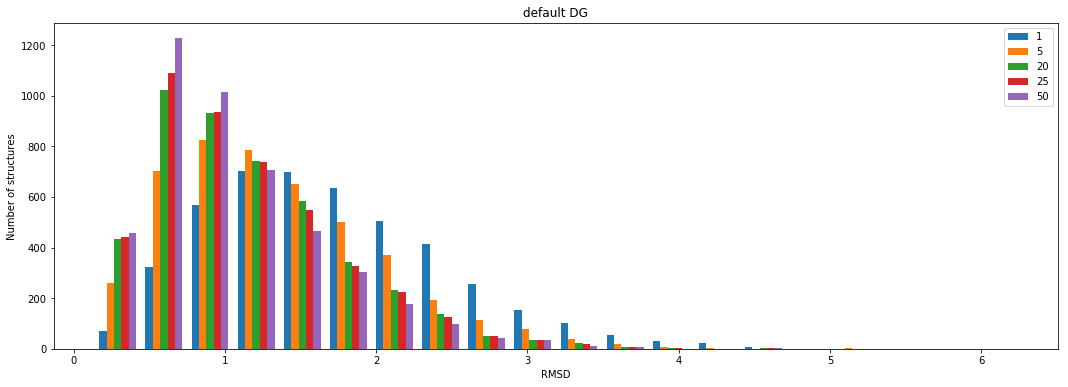Another way of looking at that is with cumulative box plots similar to what was used in Fig 7 of the ETKDG paper. These show the fraction of structures that have a minimum RMSD less than a particular value.
In :
```def fig7_plot(rmsvs,nmols,title,blocks=(0.1,0.5,1.0,1.5,2.0)):
pltVals=[]
for thresh in blocks:
tVals = []
for k in sorted(rmsvs):
tVals.append(len([1 for x in rmsvs[k] if x<thresh])/nmols)
pltVals.append(tVals)
N = len(rmsvs.keys())
ind = np.arange(N)
w=0.1
figsize(18,6)
fig,ax = subplots()
rects=[]
for i,nc in enumerate(rmsvs):
rects.append(ax.bar(ind+i*w,[x[i] for x in pltVals],w))
ax.set_xticks(ind+(w*len(blocks)/2))
ax.set_xticklabels('<%.2f'%x for x in blocks)
ax.set_ylabel('Fraction of structures')
ax.set_xlabel('RMSD')
ax.set_title(title)
ax.legend((r for r in rects),('%d'%k for k in sorted(rmsvs)))
ax.set_ybound(lower=0,upper=1)
ax.grid(axis='y')
```
In :
```fig7_plot(rmsvs,len(ms),'ETKDG');
```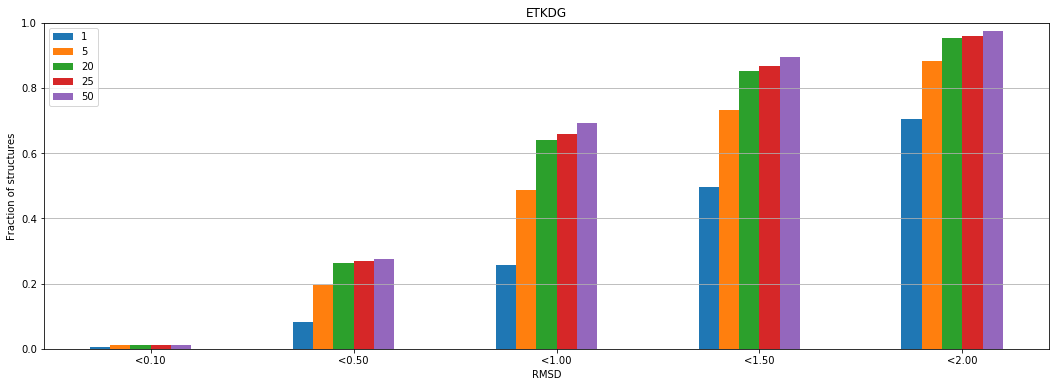In :
```fig7_plot(rmsvs2,len(ms),'DG');
```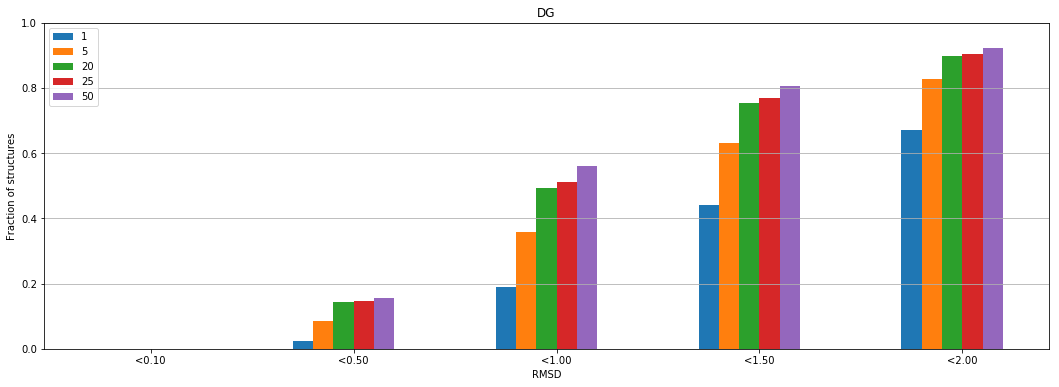Those show what we'd expect: ETKDG is significantly better than DG and adding conformations tends to help. With 50 conformations ETKDG finds a result within 1.0A of the crystal structure for about 70% of the structures.
David Koes made some interesting plots that looked at average RMSD in structures as a function of the number of rotatable bonds. Let's try a few of those.
In :
```nrots = [AllChem.CalcNumRotatableBonds(x) for x in ms_noh]
rms_by_nrot = defaultdict(lambda:defaultdict(list))
rms_by_nrot2 = defaultdict(lambda:defaultdict(list))
for i,nrot in enumerate(nrots):
for k in rmsvs:
rms_by_nrot[k][nrot].append(rmsvs[k][i])
rms_by_nrot2[k][nrot].append(rmsvs2[k][i])
```
In :
```import numpy as np
counts = sorted([(x,len(values_there(rms_by_nrot[x]))) for x in rms_by_nrot])
```
In :
```figsize(8,8)
fig, ax1 = plt.subplots()
ax2 = ax1.twinx()

# integral = [counts]
# for i in range(1,len(counts)):
#     integral.append(counts[i]+integral[-1])
# integral = [x/len(ms_noh) for x in integral]
# ax2.plot([x for x,y in counts],[y for y in integral],linestyle='--',color='r')
# ax2.set_ylabel('frac structs')

ax2.plot([x for x,y in counts],[y for x,y in counts],linestyle='--', color='r')
ax2.set_ylabel('num structs')

for k in sorted(rms_by_nrot):
means = [(x,np.average(values_there(rms_by_nrot[k][x]))) for x,_ in counts]
means = sorted(means)
ax1.plot([x for x,y in means],[y for x,y in means],label="ETKDG"+str(k))
for k in (5,50):
means = [(x,np.average(values_there(rms_by_nrot2[k][x]))) for x,_ in counts]
means = sorted(means)
ax1.plot([x for x,y in means],[y for x,y in means],linestyle=':',label="DG"+str(k))

ax1.set_ylabel('mean(RMSD)');
ax1.set_xlabel('num_rotatable')
ax1.legend();
ax2.grid(axis='y')
ax1.grid(axis='x')
```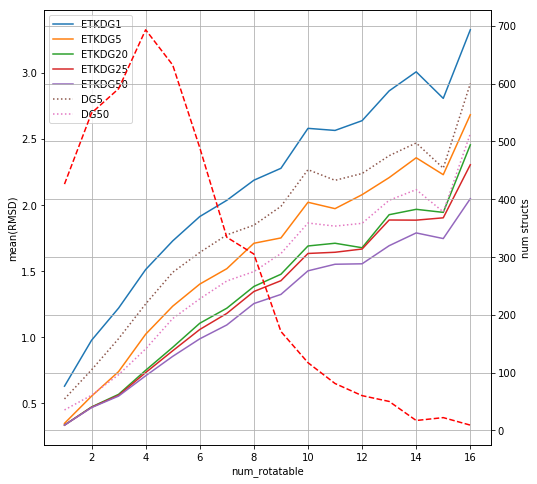I've deviated a bit from the way David presents this by adding a line showing the number of structures in the dataset with the corresponding number of rotatable bonds (the red dashed line and the right-hand Y axis). There aren't a lot of structures with more than 10 rotatable bonds in the dataset.
From the graph we can see the expected result: mean(RMSD) increases steadily with the number of rotatable bonds and ETKDG is consistently better than DG.

# Timing information¶

We know that the ETKDG procedure is more computationally intensive than DG. Let's look at how much of a difference it makes on this data set:
In :
```figsize(8,8)
ptimes = [x for i,x in enumerate(ts) if tms[i].GetNumConformers()]
ptimes2 = [x for i,x in enumerate(ts2) if tms[i].GetNumConformers()]
scatter(ptimes2,ptimes)
plot((0,12),(0,12),'r')
A = np.vstack([ptimes2, np.ones(len(ptimes2))]).T
m, c = np.linalg.lstsq(A, ptimes)
print(m,c)
grid()
plot(ptimes2,m*np.array(ptimes2)+c,color='r',linestyle='--')
xlabel('Time-DG (s)');
ylabel('Time-ETKDG (s)');
```
```1.73472205238 0.0968224468535
```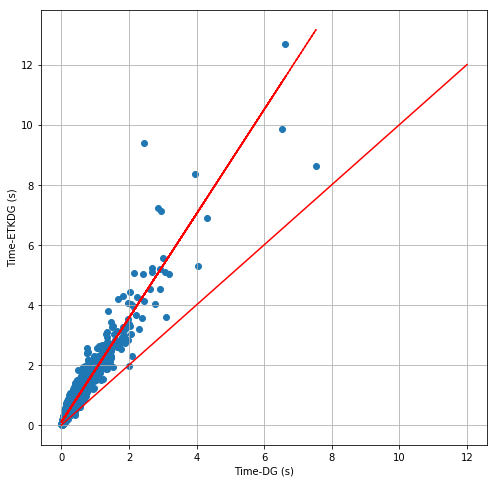So ETKDG takes, on average, 1.7 times as long.
A different look at timing: The fraction of structures that are completed in less than given time.
In :
```time_integration = defaultdict(int)
time_integration2 = defaultdict(int)
for tv in (0.1,.25, .5, 1, 1.5, 2, 3, 5,10,20):
time_integration[tv] = len([1 for x in ts if x<=tv])
time_integration2[tv] = len([1 for x in ts2 if x<=tv])
figsize(8,8)
plot(sorted(time_integration),[time_integration[x]/len(ms_noh) for x in sorted(time_integration)],label='ETKDG')
plot(sorted(time_integration),[time_integration2[x]/len(ms_noh) for x in sorted(time_integration)],label='DG')
grid()
xlabel('time (s)')
ylabel('fraction of structures')
legend()
xscale('log');
```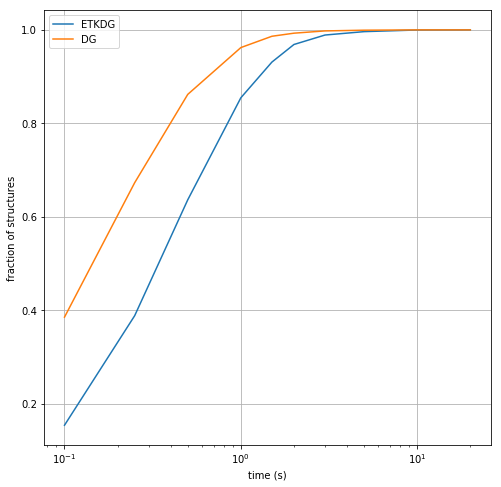Interpretation of this: both ETKDG and DG are able to generate conformations (remember that the target number was 50 conformations with an RMS threshold of 0.5A between them) for >80% of the structures in less than 1 second. ETKDG completes ~85% and DG completes about 95%.
Look at how sensitive those times are to the number of rotatable bonds:
In :
```times_by_nrot = defaultdict(list)
times_by_nrot2 = defaultdict(list)
for i,nrot in enumerate(nrots):
if not tms[i].GetNumConformers(): continue
times_by_nrot[nrot].append(ts[i])
times_by_nrot2[nrot].append(ts2[i])
```
In :
```figsize(8,8)
fig, ax1 = plt.subplots()
ax2 = ax1.twinx()

ax2.plot([x for x,y in counts],[y for x,y in counts],linestyle='--', color='r')
ax2.set_ylabel('num structs')

means = [(x,np.average(times_by_nrot[x]),np.std(times_by_nrot[x])) for x,_ in counts]
means = sorted(means)
ax1.errorbar([x for x,y,z in means],[y for x,y,z in means],yerr=[z for x,y,z in means],label="ETKDG-50")
means = [(x,np.average(times_by_nrot2[x]),np.std(times_by_nrot2[x])) for x,_ in counts]
means = sorted(means)
ax1.errorbar([x for x,y,z in means],[y for x,y,z in means],yerr=[z for x,y,z in means],label="DG-50")

ax1.set_ylabel('time (s)');
ax1.set_xlabel('num_rotatable')
ax1.legend();
ax2.grid(axis='y')
ax1.grid(axis='x')
ax1.set_ybound(0,3)
```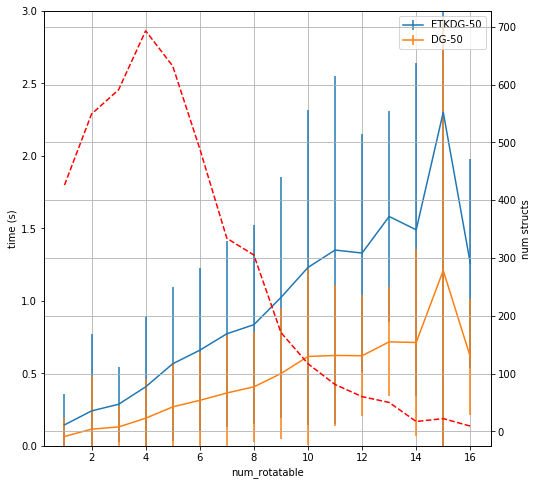Adding rotatable bonds causes conformation generation to take longer. This isn't a huge surprise.

# Impact of force-field minimization¶

David also does an analysis of the impact of UFF minimization on the RMSD values. I'm not going to repeat exactly that, but it is worth seeing if minimizing the conformations improves the RMSDs. We'll do that here with UFF.
In :
```minms = [Chem.Mol(m) for m in tms]
mintimes = []
for i,m in enumerate(minms):
t1 = time.time()
if not m.GetNumConformers():
continue
AllChem.UFFOptimizeMoleculeConfs(m,numThreads=0)
t2 = time.time()
mintimes.append(t2-t1)
if not i%500:
print("Done:",i)
```
```Done: 0
```
```RDKit ERROR: [05:11:24] UFFTYPER: Unrecognized charge state for atom: 14
```
```Done: 500
```
` ...`
`Done: 4000`
```RDKit ERROR: [05:26:56] UFFTYPER: Unrecognized charge state for atom: 13
RDKit ERROR: [05:27:20] UFFTYPER: Unrecognized charge state for atom: 12
```
```Done: 4500
```
In :
```minms_noh = [Chem.RemoveHs(m) for m in minms]
```
In :
```with open('../data/platinum_dataset_2017_01.chiral_confs.minimized.pkl','wb+') as outf:
pickle.dump((minms_noh,mintimes),outf)
```
In :
```rmsvs_min = defaultdict(list)
for m,tm in zip(ms_noh,minms_noh):
best = 1e8
cids = [x.GetId() for x in tm.GetConformers()]
for i,cid in enumerate(cids):
rms = AllChem.GetBestRMS(m,tm,probeConfId=cid)
best = min(rms,best)
if not i:
best_1 = best
if i<5:
best_5 = best
if i<20:
best_20 = best
if i<25:
best_25 = best
rmsvs_min.append(best)
rmsvs_min.append(best_1)
rmsvs_min.append(best_5)
rmsvs_min.append(best_20)
rmsvs_min.append(best_25)
```
In :
```rms_by_nrot_min = defaultdict(lambda:defaultdict(list))
for i,nrot in enumerate(nrots):
for k in rmsvs_min:
rms_by_nrot_min[k][nrot].append(rmsvs_min[k][i])
```
In :
```figsize(8,8)
fig, ax1 = plt.subplots()
ax2 = ax1.twinx()
ax2.plot([x for x,y in counts],[y for x,y in counts],linestyle='--', color='r')
ax2.set_ylabel('num structs')

for k in sorted(rms_by_nrot):
means = [(x,np.average(values_there(rms_by_nrot[k][x]))) for x,_ in counts]
means = sorted(means)
ax1.plot([x for x,y in means],[y for x,y in means],label="ETKDG"+str(k))
for k in (1,5,25,50):
means = [(x,np.average(values_there(rms_by_nrot_min[k][x]))) for x,_ in counts]
means = sorted(means)
ax1.plot([x for x,y in means],[y for x,y in means],linestyle=':',label="ETKDG_min"+str(k))

ax1.set_ylabel('mean(RMSD)');
ax1.set_xlabel('num_rotatable')
ax1.legend();
ax2.grid(axis='y')
ax1.grid(axis='x')
```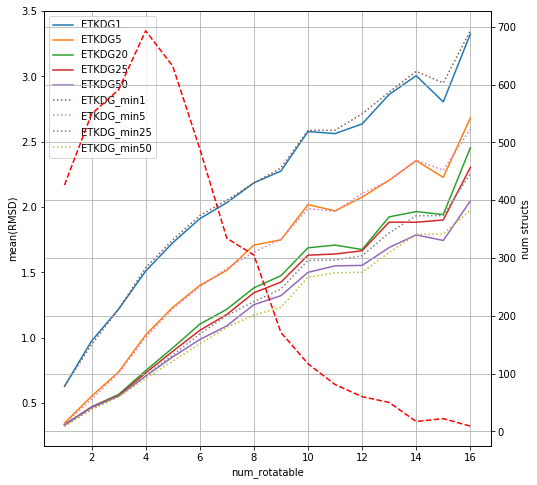The impact is not large.
There's a lot more to be looked at here with the force fields, but this blog post is already getting fairly long, so I'm going to come back to that in a future post.